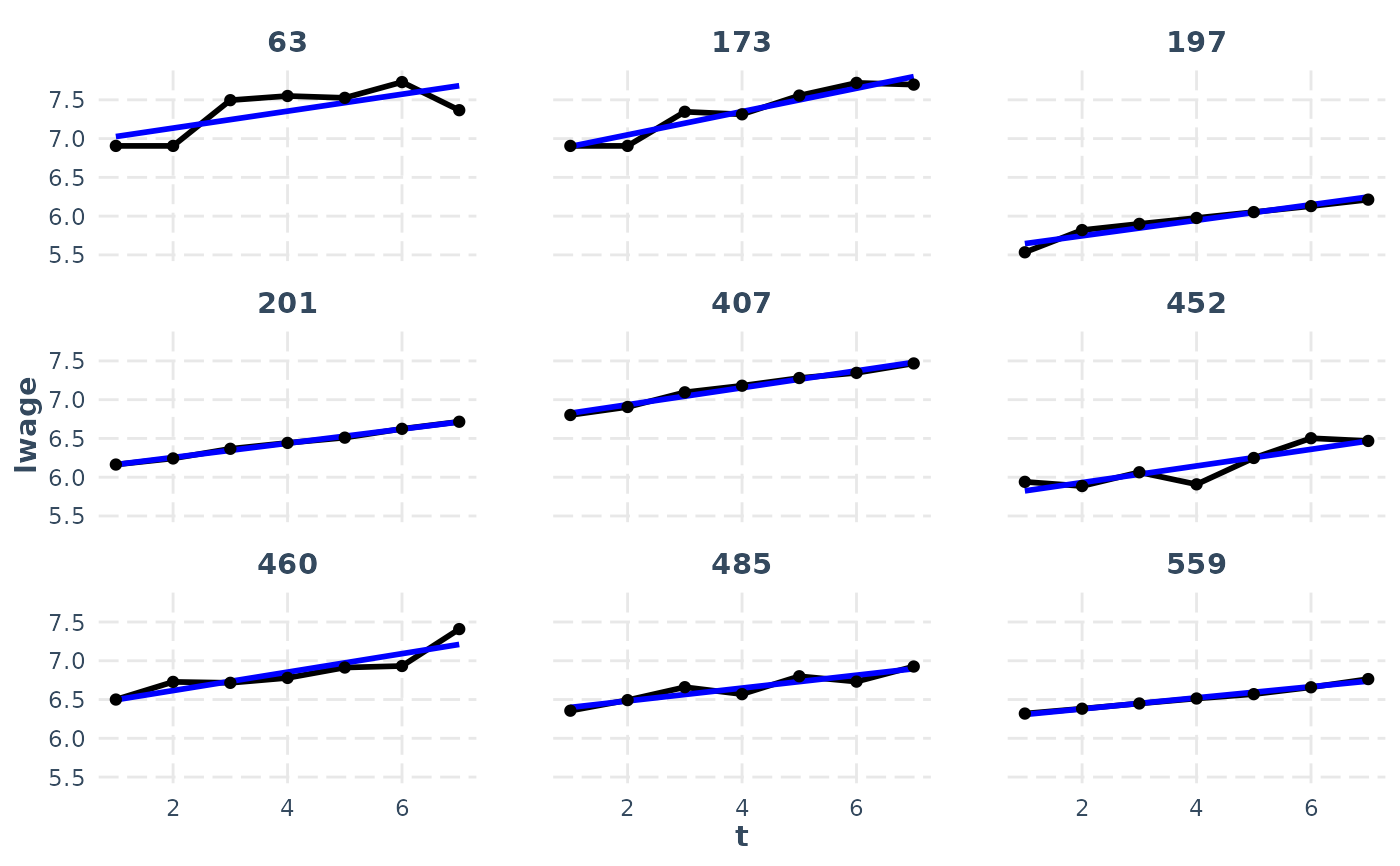line_plot allows for flexible visualization of repeated measures variables from panel_data frames.

## Usage

line_plot(
data,
var,
id = NULL,
wave = NULL,
overlay = TRUE,
show.points = TRUE,
subset.ids = FALSE,
n.random.subset = 9,
mean.function = "lm",
line.size = 1,
alpha = if (overlay) 0.5 else 1
)

## Arguments

data

Either a panel_data frame or another data frame.

var

The unquoted name of the variable of interest.

id

If data is not a panel_data object, then the id variable.

wave

If data is not a panel_data object, then the wave variable.

overlay

Should the lines be plotted in the same panel or each in their own facet/panel? Default is TRUE, meaning they are plotted in the same panel.

show.points

Plot a point at each wave? Default is TRUE.

subset.ids

Plot only a subset of the entities' lines? Default is NULL, meaning plot all ids. If TRUE, a random subset (the number defined by n.random.subset) are plotted. You may also supply a vector of ids to choose them yourself.

n.random.subset

How many entities to randomly sample when subset.ids is TRUE.

Add a line representing the mean trend? Default is FALSE. Cannot be combined with overlay.

mean.function

The mean function to supply to geom_smooth when add.mean is TRUE. Default is "lm", but another option of interest is "loess".

line.size

The thickness of the plotted lines. Default: 0.5

alpha

The transparency for the lines and points. When overlay = TRUE, it is set to 0.5, otherwise 1, which means non-transparent.

## Value

The ggplot object.

## Examples


data("WageData")
wages <- panel_data(WageData, id = id, wave = t)
line_plot(wages, lwage, add.mean = TRUE, subset.ids = TRUE, overlay = FALSE)
#> geom_smooth() using formula = 'y ~ x'